# 聊聊 C++ 中的几种智能指针 （下）

1. unique_ptr

2. shared_ptr

3. weak_ptr

## 二：三大智能指针详解

### 1. unique_ptr


void test() {
int* i = new int(10);

auto_ptr<int> ptr1(i);
auto_ptr<int> ptr2 = ptr1;  //将 ptr1 的控制台送给了 ptr2

cout << *ptr1 << endl;
}

int main() {
test();
}




class unique_ptr {

public:
unique_ptr(const unique_ptr&) = delete;
unique_ptr& operator=(const unique_ptr&) = delete;
};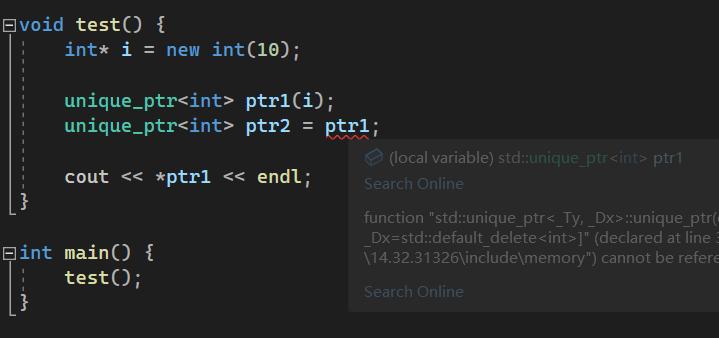### 2. shared_ptr


void test() {
int* i = new int(10);
shared_ptr<int> ptr1(i);
shared_ptr<int> ptr2 = ptr1;
shared_ptr<int> ptr3 = ptr2;

printf("ptr.refcnt= %d, ptr2.refcnt=%d, ptr3.refcnt=%d \n",
ptr1.use_count(),
ptr2.use_count(),
ptr3.use_count());
}

int main() {
test();
}class _Ref_count_base {
private:
_Atomic_counter_t _Uses = 1;
_Atomic_counter_t _Weaks = 1;
}




class shared_ptr : public _Ptr_base<_Ty> {
~shared_ptr() noexcept { // release resource
this->_Decref();
}
}

class _Ref_count_base {

void _Decref() noexcept { // decrement use count
if (_MT_DECR(_Uses) == 0) {
_Destroy();
_Decwref();
}
}
}




#include <iostream>
#include <string>

using namespace std;

class BClass;

class AClass
{
public:
int i = 10;
shared_ptr<BClass> b;
};

class BClass
{
public:
int i = 11;
shared_ptr<AClass> a;
};

void test() {

AClass* a = new AClass();
BClass* b = new BClass();

shared_ptr<AClass> aclass(a);
shared_ptr<BClass> bclass(b);

aclass->b = bclass;
bclass->a = aclass;

printf("a.refcnt=%d, b.refcnt=%d, a=%x,b=%x \n", aclass.use_count(), bclass.use_count(), &(*a), &(*b));
}

int main() {
test();
}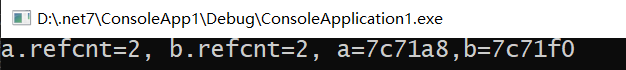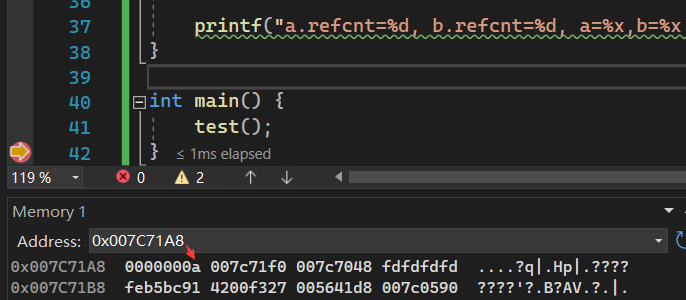### 3. weak_ptr


class AClass
{
public:
int i = 10;
weak_ptr<BClass> b;
};

class BClass
{
public:
int i = 11;
weak_ptr<AClass> a;
};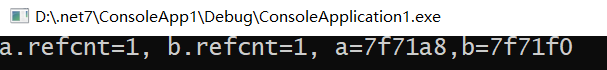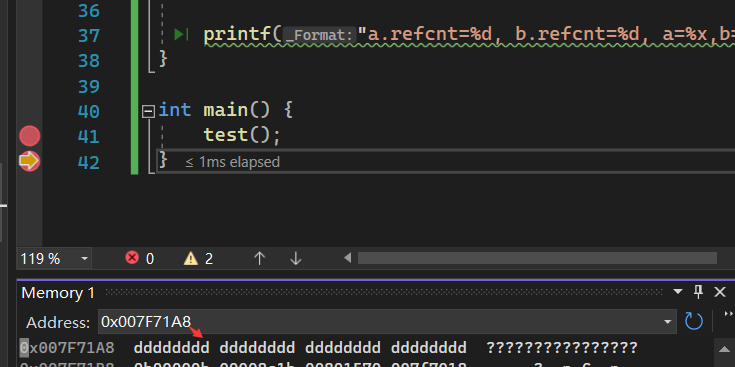posted @ 2022-07-24 11:01  一线码农  阅读(97)  评论(0编辑  收藏  举报